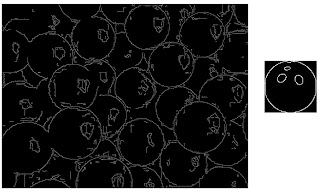Sunday, June 30, 2013

Image objects recognition: a basic approach

To complete the brief dissertation on the most popular problems of the image analysis science, I did a couple of experiments on a very intriguing topic: the identification of specific objects within an image.
I didn't use "state of art" algorithms (...my knowledge on the field is not deep and I wont use techniques that I don't fully understand!).
The target of this experiment isn't to show the best way, but just to show the rudiments of the matter.

Objective
Given an image called target image, and given another image called feature, the objective is to identify the features within the target image.

Technique
I decided to play with a technique that I studied on signal processing course at University: the matrix correlation.

The matrix correlation is typically used to detect similarities between two 2D signals, which are often saved in matrices.
The magnitude of the correlation shows how similar the signals are. If the correlation is large, the two signals are considered to be very similar. Alternatively, if correlation is zero, the two signals are considered to be independent. Analytical definition and explanation are available in all books of signal processing.
Experiment: Ball detection
In the first example I will try to detect the balls in the target image:On the left the target image. On the right the feature (the ball!) to be identified in the image.
To solve the problem, I tried to apply the algorithm directly to the original images, but the results were terrible, so I applied some image manipulation technique in order to make more digestible the input to the main algorithm: I never be tired to say that preprocessing is crucial to solve a problem!
The algorithms used here are: edge detection and binarization. So I feed the correlation algorithm with the following input:The input used to feed the correlation algorithm obtained with Binarization and eEge Detection techniques.
Results
Finally we can show the output: we can check if the algorithm identified correctly in the target image the balls!
To highlight the results I played around with colors and image merging :)Results: balls identified in the image
As you can see the results are not so bad, and playing around with a preprocessing parameters I'm sure it's possible to improve the accuracy.
Experiment: Painting Recognition
I Applied the same technique to identify the paintings hung on a wall:Target image: paintings hung on a wall
Results:
The techniques used are same of the above experiment, so let's go directly to the results:Paintings detected
Considerations
The technique is quite rough and the accuracy depends on
• Preprocessing technique applied
• Measure used to compute the correlation (in both experiment I used cosine distance)
The algorithm per se seems to be not so general purpose to be used for such objective, but it's a good  starting point to approach the problem. Maybe if the feature to be detected is always the same (for instance as in face recognition), the accuracy is acceptable.
Stay Tuned
Cristian